1594933620

# Contents: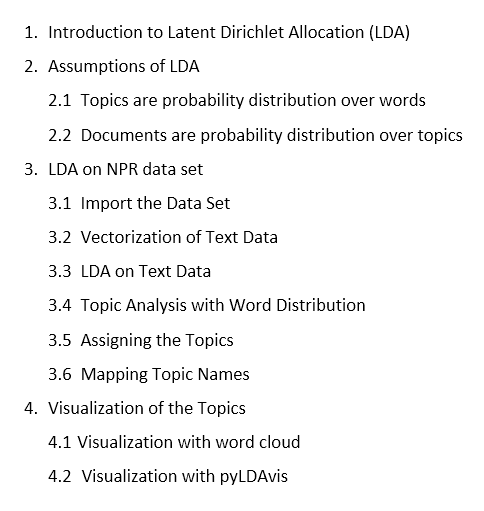# 1. Introduction to Latent Dirichlet Allocation (LDA):

LDA stands for Latent Dirichlet Allocation. As time is passing by, data is increasing exponentially. Most of the data is unstructured and a few of them are unlabeled. It is a tedious task to label each and every data manually. How can we label such a huge amount of data if not manually? Here comes the LDA to our rescue. LDA is one of the topic modeling techniques which is used to analyze a huge amount of data, cluster them into similar groups, and label each group. It should be noted that LDA technique is for unsupervised learning which is used to label the data by grouping them into similar topics. Unlike K-Means clustering and other clustering techniques which uses the concept of distance between cluster center, LDA works on the probability distribution of topics belonging to the document.

# 2. Assumptions of LDA:

## 2.1 Topics are probability distribution over words:

It represents the probability distribution of words belonging to the topics. Suppose, we have 2 topics — Healthcare and Politics. Words like medicine, injection, oxygen, etc, will have a higher probability distribution belonging to the topic of Healthcare while words like election, voting, etc, will have a lower probability distribution. On the other hand, words like election, voting, party, have a higher probability distribution belonging to the topic Politics while words like medicine, injection, etc, will have a lower probability distribution. In this, each topic shares a similar group of words with a higher probability. Refer to the below image for a clear understanding.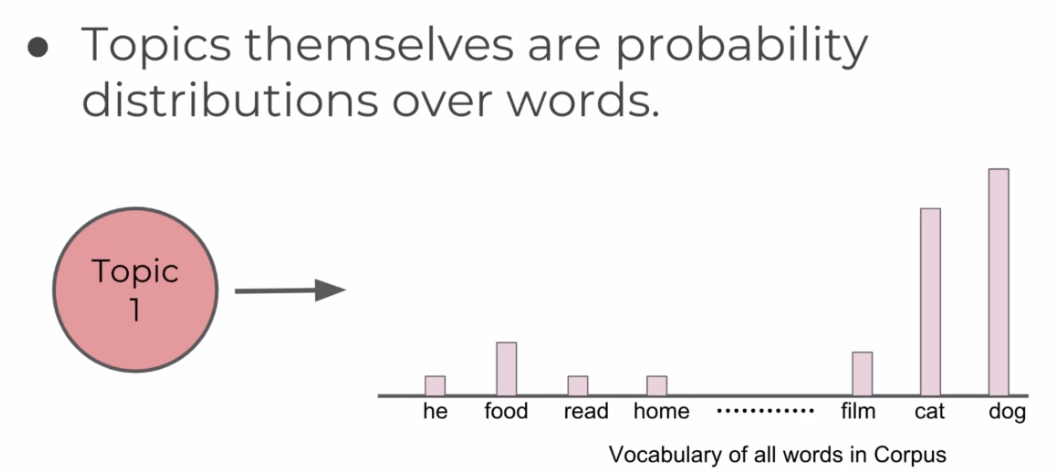## 2.2 Documents are probability distribution over topics:

It represents the probability distribution of topics belonging to each document. As in the above example, we have 2 topics — Healthcare and Politics. Since the documents are the mixture of various words, each word will have a probability distribution belonging to the topics. This results in the probability distribution of topics belong to each document. Confusing, right! Even I was confused in the beginning but, after pondering over it for some time, I was able to understand it. Let me try to explain in other terms. Consider a document that states —** “We have observed a lot of patients recovered last month. Government’s fund has increased the supply of medicine.”** If we read the statement, it sounds more relatable to the Healthcare topic than the Government. Though we have words like ‘Government’s fund’ in the document which relates to the Politics, due to its lower probability, when compared to the words like patients, recover, medicine, the document can be labeled as ‘Healthcare’. Refer to the below image for a clear understanding.

#unsupervised-learning #data analysis

## Buddha Community1595709180

## The intuition behind Latent Dirichlet Allocation (LDA)

I know LDA is used for topic modeling and also know how I have to ingest text data and what outcome I can expect. But what I didn’t get was which mathematical part is responsible for finding the latent or unknown topics and was super interested in understanding the logic to arrive at a model to fit it to the data, other logical steps involved in discovering the latent topics, and the algorithm used to optimize the solution.

My known unknowns about the topic are plenty and so I decided to write a post to hunt some of them down with clarity. After going through research papers, videos, and other sources of information, I have come to an understanding of how topics get discovered and I would like to share it with other machine-learning enthusiasts like you.

Bear with me while we take the following technical ride; will be worth it!

My questions were:

What’s Dirichlet and why use Dirichlet in LDA?

What do the different variables in the model represent?

What’s Dirichlet?

It turns out Dirichlet distribution is a generalization of beta distribution extended to multiple dimensions. What’s a beta distribution? It’s a univariate distribution of random variables that belong to the range 0 to 1 and parameterized with parameters alpha and beta. Beta is also the conjugate prior for a binomial distribution with probability, p. Then the deal is that the posterior distribution of p is also a beta distribution with parameters alpha_dash=alpha+ number of successes and beta_dash= beta+ number of failures. Likewise when we assume a Dirichlet distribution as prior, in the case of LDA, the posterior will also be a Dirichlet.

Why use Dirichlet in LDA?

A multinomial distribution is a generalization of the binomial distribution and models the outcome of n experiments, where the outcome of each trial has a categorical distribution. And it’s fitting in the current context where LDA models the outcome for D different documents, where the outcome of each document has different topics. In LDA, we want the topic mixture proportions for each document to be drawn from some distribution, preferably from a probability distribution so it sums to one. So for the current context, we want probabilities of probabilities. Therefore we want to put a prior distribution on multinomial. We pick Dirichlet because it is a conjugate prior for the multinomial distribution. If our likelihood is a multinomial with a Dirichlet prior, then the posterior is also a Dirichlet as mentioned above.

#lda #bayesian-machine-learning #machine-learning #topic-modeling #entropy1601444100

## Topic Modeling of Earnings Calls using Latent Dirichlet Allocation (LDA)

Most listed US companies host earnings calls every quarter. These are conference calls where management discusses financial performance and company updates with analysts, investors and the media. Earnings calls are important — they highlight valuable information for investors and provide an opportunity for interaction through Q&A sessions.

There are hundreds of earnings calls held each quarter, often with the release of detailed transcripts. But the sheer volume of those transcripts makes analyzing them a daunting task.

Topic modeling is a way to streamline this analysis. It’s an area of natural language processing that helps to make sense of large volumes of text data by identifying the key topics or themes within the data.

In this article, I show how to apply topic modeling to a set of earnings call transcripts. I use a popular topic modeling approach called Latent Dirichlet Allocation and implement the model using Python.

I also show how topic modeling can require some judgement, and how you can achieve better results by adjusting key parameters.

#text-analytics #naturallanguageprocessing #earnings-call #machine-learning #topic-modeling1593771600

## Topic Modeling — LDA Mallet Implementation in Python — Part 3

As you may recall, we defined a variable `tm_results` to store topic distributions for each document. `pprint`the first item of `tm_results`to see how it looks like:

``````pprint(tm_results)
``````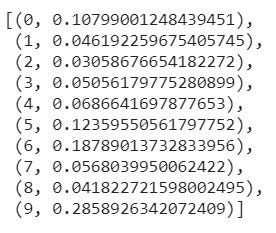As seen above, the first item is a list of tuples and you get topic distributions for the first document. Since `tm_results` store topic distributions for all the documents, we may create a dataframe to analyze the data. We can convert each record to a dictionary and then use `pandas.DataFrame.from_records` to transform `tm_results`to a dataframe:

``````df_weights = pd.DataFrame.from_records([{v: k for v, k in row} for row in tm_results])
df_weights.columns = ['Topic ' + str(i) for i in range(1,11)]
df_weights
``````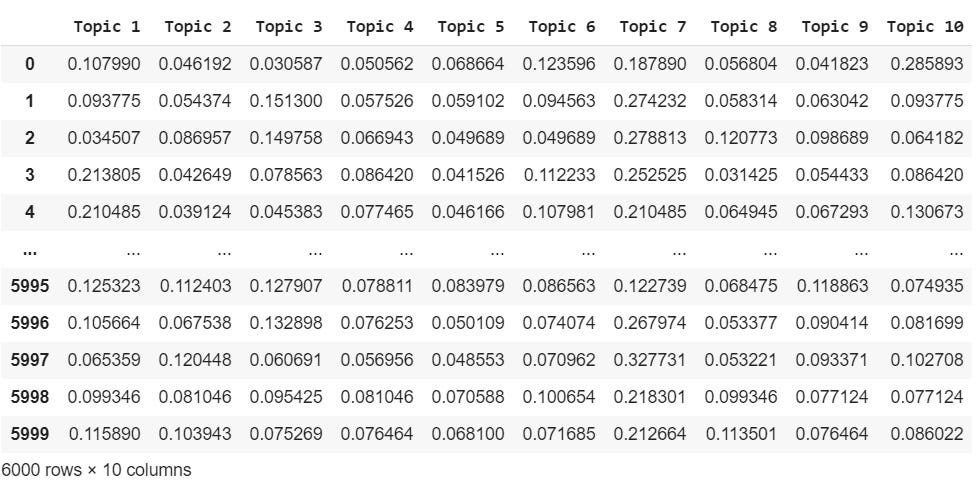We can add “Year” column and get the average of yearly topic weights:

``````df_weights['Year'] = df.Year
df_weights.groupby('Year').mean()
``````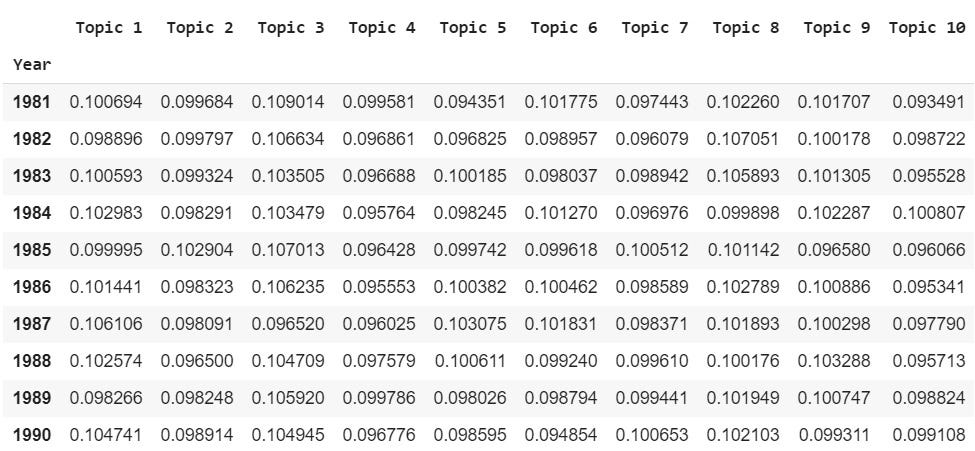As you can see the yearly average weights of topics are so close. In my experience, Mallet generally produces close probabilities among topics. Therefore, I prefer to get dominant topics for each document and then do the analysis based on dominant topics.

To get which topic is dominant in each document we can use `pandas.DataFrame.idxmax()` function. It returns the index of maximum value over a requested axis. But first, we need to drop ‘Year’ column and left only 10 topics as columns. Then, we can get column index of maximum value for each row and assign the values to ‘Dominant’ topic.

``````df_weights['Dominant'] = df_weights.drop('Year', axis=1).idxmax(axis=1)
``````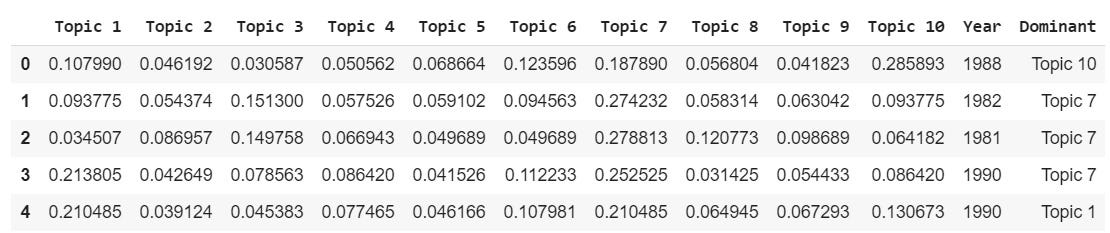Now, we can get the percentage of dominant topics in a given year by grouping the dataframe by ‘Year’ column and call `value_counts(normalize=True)` function on ‘Dominant’ column. When we perform the functions so far, we get a multi-index pandas Series. To convert it to a dataframe where rows are “years” and columns are “topics”, we need to chain `unstack()`function.

``````df_dominance = df_weights.groupby('Year')['Dominant'].value_counts(normalize=True).unstack()
df_dominance
``````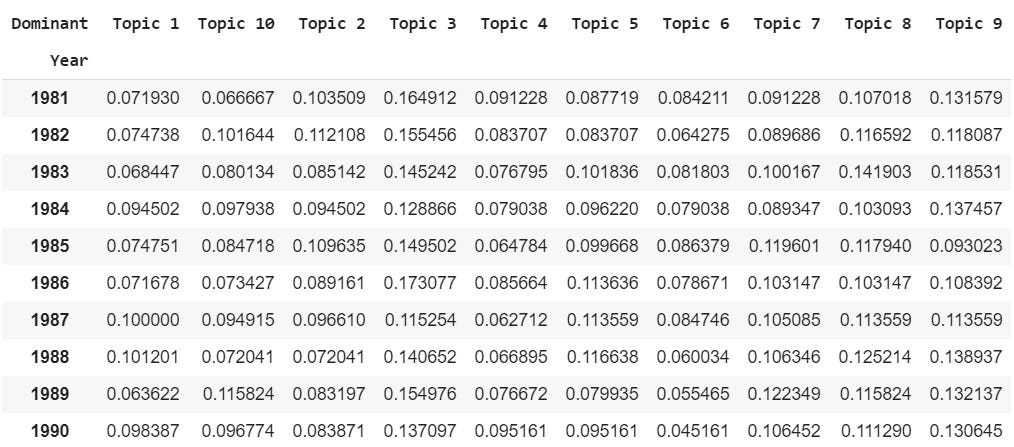As you can see from the above output, the trends are more clear now. We can also get trends for each “journal”. First, we add “Journal” column to `df_weights` dataframe and perform similar actions that we did above.

``````df_journals = df_weights.groupby(['Journal', 'Year'])['Dominant'].value_counts(normalize=True).unstack()
``````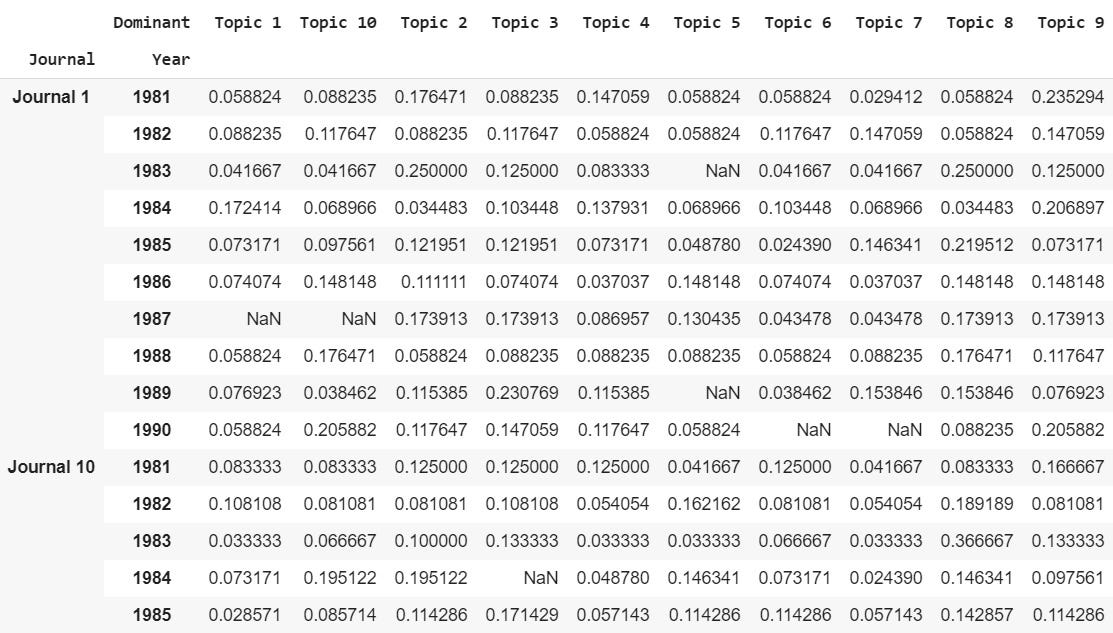#pandas #mallet #lda #python #topic-modeling1593764280

## Topic Modeling — LDA Mallet Implementation in Python — Part 2

We should define path to the mallet binary to pass in LdaMallet wrapper:

``````mallet_path = ‘/content/mallet-2.0.8/bin/mallet’
``````

There is just one thing left to build our model. We should specify the number of topics in advance. Although there isn’t an exact method to decide the number of topics, in the last section we will compare models that have different number of topics based on their coherence scores.

For now, build the model for 10 topics (this may take some time based on your corpus):

``````ldamallet = gensim.models.wrappers.LdaMallet(mallet_path, corpus=corpus, num_topics=10, id2word=id2word)
``````

Let’s display the 10 topics formed by the model. For each topic, we will print (use pretty print for a better view) 10 terms and their relative weights next to it in descending order.

``````from pprint import pprint
# display topics
pprint(ldamallet.show_topics(formatted=False))
``````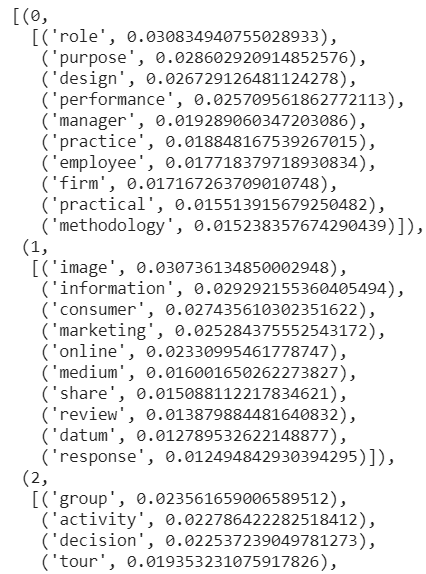Note that, the model returns only clustered terms not the labels for those clusters. We are required to label topics.

We can calculate the coherence score of the model to compare it with others.

``````# Compute Coherence Score
coherence_model_ldamallet = CoherenceModel(model=ldamallet, texts=data_ready, dictionary=id2word, coherence='c_v')

coherence_ldamallet = coherence_model_ldamallet.get_coherence()
print('Coherence Score: ', coherence_ldamallet)
``````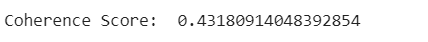It’s a good practice to pickle our model for later use.

``````import pickle
pickle.dump(ldamallet, open("drive/My Drive/ldamallet.pkl", "wb"))
``````

You can load the pickle file as below:

``````ldamallet = pickle.load(open("drive/My Drive/ldamallet.pkl", "rb"))
``````

We can get the topic modeling results (distribution of topics for each document) if we pass in the corpus to the model. You can also pass in a specific document; for example, `ldamallet[corpus]` returns topic distributions for the first document. For the whole documents, we write:

``````tm_results = ldamallet[corpus]
``````

We can get the most dominant topic of each document as below:

``````corpus_topics = [sorted(topics, key=lambda record: -record) for topics in tm_results]
``````

To get most probable words for the given topicid, we can use `show_topic()` method. It returns sequence of probable words, as a list of (word, word_probability) for specific topic. You can get top 20 significant terms and their probabilities for each topic as below:

``````topics = [[(term, round(wt, 3)) for term, wt in ldamallet.show_topic(n, topn=20)] for n in range(0, ldamallet.num_topics)]
``````

#mallet #lda #pandas #topic-modeling #python1593945180

# How to Use Latent Dirichlet Allocation (LDA)?

In here, I won’t cover the mathematical foundation of the LDA. I will just discuss how to interpret the results of LDA topic modeling.

During LDA Topic modeling, we create many different topic groups. As a researcher, we are the one who decides the number of groups in the output. However, we do not know what is the best number of groups. Therefore, we will obtain different numbers of groups. Then, we examine and compare topic modelings, and decide which topic model makes more sense, most meaningful, have the clearest distinction within the model. Then, the group (model) that makes the most sense will be chosen among all topic groups.

It must be noted that the nature of the LDA is subjective. It’s possible that different people may reach different conclusions about choosing the most meaningful topic groups. We are looking for the most reasonable topic groups. People from different backgrounds, different domain expertise may not be on the same page about most sensible topic groups.

LDA is an unsupervised clustering method. When we are talking about unsupervised clustering methods, we need to mention K-Means Clustering. K-Means Clustering is one of the most well-known unsupervised clustering methods. It’s very practical and useful in many cases and has used for text mining for years. In contrast to K-means clustering, where each word can only belong to one cluster (hard-clustering), LDA allows for ‘fuzzy’ memberships (soft clustering). Soft-clustering allows overlap among the cluster, whereas, in hard-clustering, clusters are mutually exclusive. What does this mean? In LDA, a word can belong to more than one group while this is not possible in K-Means clustering. In LDA, this trade-off makes it easier to find similarities between the words. However, this soft-clustering makes it hard to obtain distinct groups because the same words can appear in different groups. We will experience this drawback in our further analysis.

Once the topic modeling technique is applied, the researcher’s job as a human is to interpret the results and see if the mix of words in each topic makes sense. If they don’t make sense, we can try changing up the number of topics, the terms in the document-term matrix, model parameters, or even try a different model.

# Preparation of the data

**Brief information about the data I use: **The data used in this study were downloaded from Kaggle. It was uploaded by the Stanford Network Analysis Project. The original data is coming from the study of ‘From amateurs to connoisseurs: modeling the evolution of user expertise through online reviews’ done by J. McAuley and J. Leskovec (2013). This data set consists of reviews of fine foods from Amazon. The data includes all 568,454 reviews spanning 1999 to 2012. Reviews include product and user information, ratings, and a plain text review.

In this study, I will focus on ‘good reviews’ on Amazon. I defined ‘good reviews’ as the reviews that have 4-star or 5-star customer reviews (out of 5). In other words, if an Amazon review is 4 or 5 stars, it’s called ‘good review’ in this study. 1,2 or 3-star reviews are labeled as ‘bad reviews’.

Data preparation is a critical part because if we fail to prepare the data properly, we can’t perform topic modeling. Here, I won’t dive into detail because data preparation is not the focus of this study. However, be ready to spend some time here in case you have a problem. If you adjust the codes I provide here for your own dataset, I hope you should not have any problem.

#python #topic-modeling #machine-learning #data-science